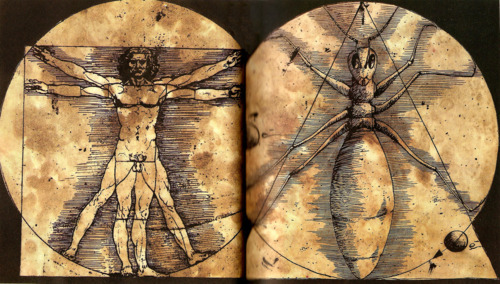Posted in Science & Nature

Ant Sequence

Find the rule for the following sequence of numbers:

1
11
21
1211
111221
312211
13112221
1113213211The solution to this sequence, which appears to not have a logical, mathematical rule, is rather simple. It is formed from grouping repeating numbers by reading them aloud, forming the next number.

This is easier to show using the above sequence as an example:

The first number is “one 1”, so the next number is 11. The second number is “two 1s”, so the next number is 21. The third number is “one 2, one 1”, so the next number is 1211. Using this rule, the sequence continues infinitely.

Interestingly, this sequence only uses the numbers 1, 2 and 3 (unless the initial number is 4 or more repeating numbers). The only number that the sequence does not work on is 22 (22, 22, 22, …).
If the initial number is k, which is between 2 and 9, then the following sequence is always produced:

k, 1k, 111k, 311k, 13211k, 111312211k, 31131122211k, …

The official name of this sequence is the Look-and-say sequence, but as it is prominently featured in Bernard Werber’s novel, The Revolution of the Ants, it is also known as the Ant sequence.